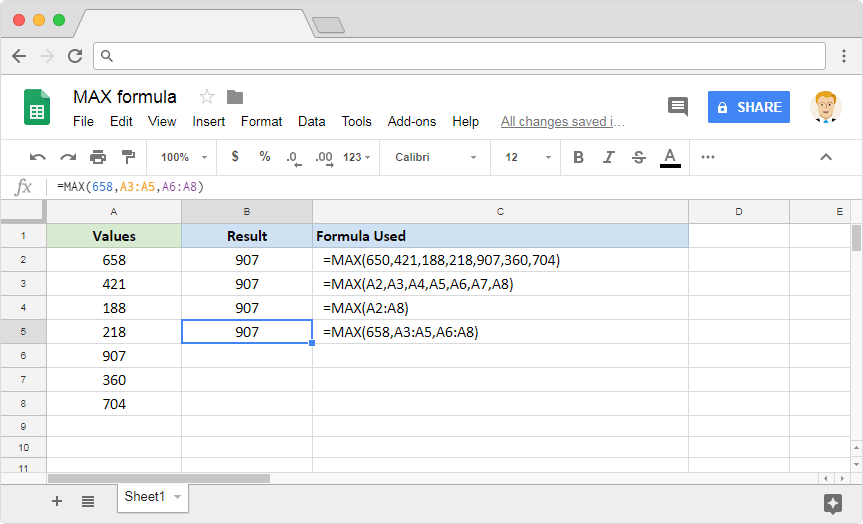# How to use the MAX formula in Google SheetsVarious spreadsheets programs that we have been using have one main goal. To help us understand the underlying data. More specifically with numbers. We often find ourselves in situations where we need to identify the maximum value from a set of numeric values. Is there any way to do this swiftly, without having to do the scouting manually? Of course! The MAX formula in Google Sheets is built just for this purpose.

### Syntax

MAX(value1, [value2, …])

• value1 – is the first input value in the set of numeric values, which the formula has to identify the maximum value from.
• value2, … – [ OPTIONAL ] – these are additional values in the set of numeric values we are considering to calculate the maximum value from.

Please note that these inputs can either be direct numeric values, or references to the cells having these numeric values. It is also possible to have direct values for a few parameters and ranges for the other parameters.

### Usage: MAX formula in Google Sheets

Now that we’ve learned the syntax of the formula, let us try it directly on the Google Sheets application. Please consider the image below.The first example consumes direct numeric values and returns the maximum value out of them. The second example is a demonstration that shows us that the MAX formula can use references to cells that store numbers. In the third example, instead of sending multiple values or references, we have passed a single composite range. This composite cell range consists of all the values from A2 through A8. And, in the final example, we not only passed direct numeric values but also a couple range references.

You’ll observe that the output is the same across all examples, regardless of the approach that we have taken with the formula. We will see that, of all the numbers we used for the input parameters, 907 is the largest value. Therefore, the MAX formula has diligently delivered what it was set out to do.

The MIN formula in Google Sheets identifies the minimum value from a set of numeric values. Read about it in this blog post.